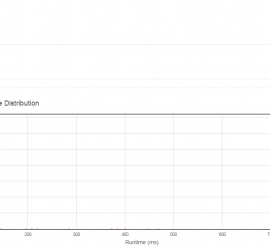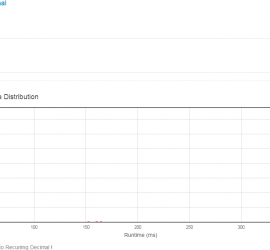# Daily Archives: September 5, 2015

## [leetcode] Majority Element

Majority Element Given an array of size n, find the majority element. The majority element is the element that appears more than ⌊ n/2 ⌋ times. You may assume that the array is non-empty and the majority element always exist in the array. Credits: Special thanks to @ts for adding […]## [leetcode] Fraction to Recurring Decimal

Fraction to Recurring Decimal Given two integers representing the numerator and denominator of a fraction, return the fraction in string format. If the fractional part is repeating, enclose the repeating part in parentheses. For example, Given numerator = 1, denominator = 2, return “0.5”. Given numerator = 2, denominator = […]